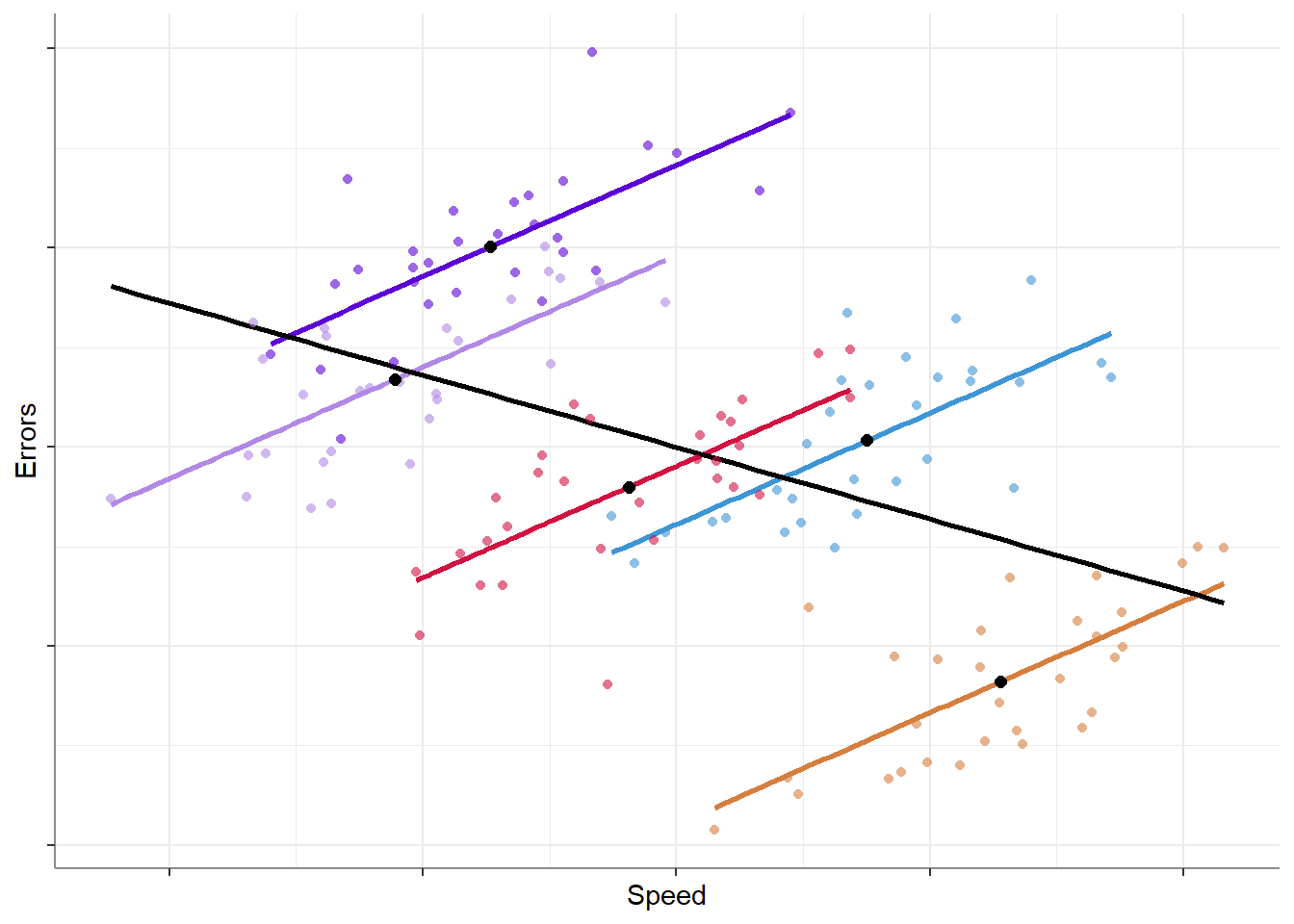# Accounting for Within- AND Between-Subject Effects

statistics
mixed-design
code
linear models
Author

Mattan S. Ben-Shachar

Published

October 21, 2019

This is a comment on Solomon Kurz’s (@SolomonKurz) recent post1 where he discusses how group-level data does not always reflect individual-level processes. I highly recommend reading his series of posts on the topic!

In this post I will demonstrate:

1. How to model both group-level processes and individual-level processes from individual-level data, using linear-mixed models.
2. When you shouldn’t worry about group-level processes differing from individual-level processes. (controlled experiments for the win!)

## Modeling individual- and group-level effects

Let’s work with the now-classic typing speed example. We take a group of 5 typists, and measure the speed of their typing (words per minute), and the rate of typing errors (errors per 100-words). Looking at the data we might get something like this:

```````geom_smooth()` using formula = 'y ~ x'
`geom_smooth()` using formula = 'y ~ x'``````As we can see, we have two sources of variation that can be used to explain or predict the rate of errors:

1. Overall, faster typists make less mistakes (group-level pattern).
2. When typing faster, typists make more mistakes (individual-level pattern).

We can model these using liner mixed models, but first we need to split our predictor (speed) into two variables, each representing a different source of variance - each typist’s average typing speed, and the deviation of each measurement from the typist’s overall mean:2

``````library(dplyr)
data <- data %>%
group_by(ID) %>%
mutate(speed_M = mean(speed),
speed_E = speed - speed_M) %>%
ungroup()

``````# A tibble: 6 × 5
ID  speed errors speed_M speed_E
<int>  <dbl>  <dbl>   <dbl>   <dbl>
1     1 -0.773 -1.74   -0.188 -0.585
2     1 -0.144 -0.703  -0.188  0.0438
3     1 -0.686 -1.73   -0.188 -0.498
4     1  0.560  1.17   -0.188  0.748
5     1  0.214  0.316  -0.188  0.402
6     1  0.179  0.392  -0.188  0.367 ``````

Let’s fit a liner mixed model and see how we can detect both patterns correctly.

``````library(lmerTest)

fit <- lmer(errors ~ speed_M + speed_E + (1 + speed_E | ID),
data = data)``````
``````Your model may suffer from singularity (see see `?lme4::isSingular` and
`?performance::check_singularity`).
Some of the standard errors and confidence intervals of the random
effects parameters are probably not meaningful!``````
Parameter Coefficient SE CI CI_low CI_high t df_error p Effects Group
(Intercept) 0.000 0.620 0.95 -1.225 1.225 0.000 143 1.000 fixed
speed_M -1.600 0.693 0.95 -2.969 -0.231 -2.309 143 0.022 fixed
speed_E 1.400 0.119 0.95 1.165 1.635 11.762 143 0.000 fixed
SD (Intercept) 1.379 0.568 0.95 0.615 3.093 NA NA NA random ID
SD (speed_E) 0.000 49027.294 0.95 0.000 Inf NA NA NA random ID
Cor (Intercept~speed_E) 1.000 297941.433 0.95 NaN 1.000 NA NA NA random ID
SD (Observations) 0.717 0.043 0.95 0.637 0.806 NA NA NA random Residual

As we can see, the slope for `speed_M` is negative (-1.6), reflecting the group-level pattern where typists who are overall faster have fewer errors; whereas the slope for `speed_E` is positive (1.4), reflecting the individual-level pattern where faster typing leads to more errors.

## When are individual-level the same as group-level patterns?

Experiments!
Or to be more precise, when we control the values of the independent variable. Why is this so? Because we control the values of the independent variable, the independent variable cannot be split into different sources of variance: there is either variance between subjects (the variable is manipulated in a between-subjects design) or there is variance within subjects (the variable is manipulated in a within-subjects design), but never both. Thus, although there can be huge heterogeneity in the way subjects present an effect, the average individual-level effect will be the same as the group-level effect (depending on the design).3

## Footnotes

1. and the short twitter discussion that followed.↩︎

2. Read more in: Hoffman, L. (2015). Time-varying predictors in models of within-person fluctuation. In Longitudinal analysis: Modeling within-person fluctuation and change (pp. 327-392). Routledge.↩︎

3. Ignoring any differences or artifacts that may arise from the differences in the design itself, such as order effects, etc.↩︎